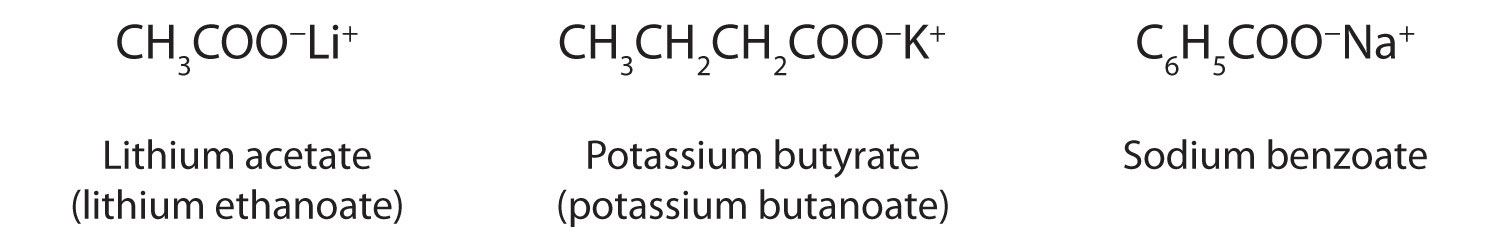# 15.5: Chemical Properties of Carboxylic Acids: Ionization and Neutralization

Skills to Develop

• Name the typical reactions that take place with carboxylic acids.
• Describe how carboxylic acids react with basic compounds.

Water-soluble carboxylic acids ionize slightly in water to form moderately acidic solutions.

$$\mathrm{RCOOH+H_2O\rightleftharpoons RCOO^-+H_3O^+}$$

Their aqueous solutions exhibit the typical properties of acids, such as changing litmus from blue to red.

The anion formed when a carboxylic acid dissociates is called the carboxylate anion (RCOO).

Whether soluble in water or not, carboxylic acids react with aqueous solutions of sodium hydroxide (NaOH), sodium carbonate (Na2CO3), and sodium bicarbonate (NaHCO3) to form salts:

RCOOH + NaOH(aq) → RCOONa+(aq) + H2O

2RCOOH + Na2CO3(aq) → 2RCOONa+(aq) + H2O + CO2(g)

RCOOH + NaHCO3(aq) → RCOONa+(aq) + H2O + CO2(g)

In these reactions, the carboxylic acids act like inorganic acids: they neutralize basic compounds. With solutions of carbonate (CO3) and bicarbonate (HCO3) ions, they also form carbon dioxide gas.

Carboxylic acid salts are named in the same manner as inorganic salts: the name of the cation is followed by the name of the organic anion. The name of the anion is obtained by dropping the -ic ending of the acid name and replacing it with the suffix -ate. This rule applies whether we are using common names or International Union of Pure and Applied Chemistry (IUPAC) names:The salts of long-chain carboxylic acids are called soaps. We discuss the chemistry of soaps elsewhere.Example $$\PageIndex{1}$$

Write an equation for each reaction.

1. the ionization of propionic acid in water (H2O)
2. the neutralization of propionic acid with aqueous sodium hydroxide (NaOH)

SOLUTION

Propionic acid has three carbon atoms, so its formula is CH2CH2COOH.

1. Propionic acid ionizes in water to form a propionate ion and a hydronium (H3O+) ion.

CH3CH2COOH(aq) + H2O(ℓ) → CH3CH2COO(aq) + H3O+(aq)
2. Propionic acid reacts with NaOH(aq) to form sodium propionate and water.

CH3CH2COOH(aq) + NaOH(aq) → CH3CH2COONa+(aq) + H2O(ℓ)

Exercise $$\PageIndex{1}$$

Write an equation for each reaction.

1. the ionization of formic acid in water
2. the ionization of p-chlorobenzoic acid in water

Example $$\PageIndex{2}$$

Write an equation for the reaction of decanoic acid with each compound.

1. aqueous sodium hydoxide (NaOH)
2. aqueous sodium bicarbonate (NaHCO3)

SOLUTION

1. Decanoic acid has 10 carbon atoms. It reacts with NaOH to form a salt and water (H2O).

CH3(CH2)8COOH + NaOH(aq) → CH3(CH2)8COONa+(aq) + H2O(ℓ)
2. With NaHCO3, the products are a salt, H2O, and carbon dioxide (CO2).

CH3(CH2)8COOH + NaHCO3(aq) → CH3(CH2)8COONa+(aq) + H2O(ℓ) + CO2(g)

Exercise $$\PageIndex{3}$$

Write an equation for the reaction of benzoic acid with each compound.

1. aqueous sodium hydroxide (NaOH)
2. aqueous sodium bicarbonate (NaHCO3)

## To Your Health: Organic Salts as Preservatives

Some organic salts are used as preservatives in food products. They prevent spoilage by inhibiting the growth of bacteria and fungi. Calcium and sodium propionate, for example, are added to processed cheese and bakery goods; sodium benzoate is added to cider, jellies, pickles, and syrups; and sodium sorbate and potassium sorbate are added to fruit juices, sauerkraut, soft drinks, and wine. Look for them on ingredient labels the next time you shop for groceries.## Concept Review Exercises

1. How does the neutralization of a carboxylic acid differ from that of an inorganic acid? How are they similar?
2. What products are formed when a carboxylic acid is neutralized with a strong base? What additional product is formed when a carboxylic acid is neutralized with a carbonate or a bicarbonate?

1. Insoluble carboxylic acids often form soluble carboxylate salts. Both form a salt and water.
2. a carboxylate salt and water; carbon dioxide

## Key Takeaways

• Soluble carboxylic acids are weak acids in aqueous solutions.
• Carboxylic acids neutralize bases to form salts.

## Exercises

1. Write the equation for the ionization of CH3CH2CH2COOH in water.

2. Write the equation for the neutralization of CH3CH2CH2COOH with sodium hydroxide [NaOH(aq)].

3. Write the equation for the reaction of CH3COOH with sodium carbonate [Na2CO3(aq)].

4. Write the equation for the reaction of CH3CH2COOH with sodium bicarbonate [NaHCO3(aq)].

5. Write the equation for the ionization of propionic acid in water.

6. Write the equation for the ionization of γ-chloropentanoic acid in water.

7. Write an equation for the reaction of butyric acid with each compound.

1. aqueous NaOH
2. aqueous NaHCO3
8. Write the condensed structural formula for each compound.

1. potassium acetate
2. calcium propanoate
9. Name each compound.

1. CH3CH2CH2COOLi+
2. CH3CH2CH2COONH4+